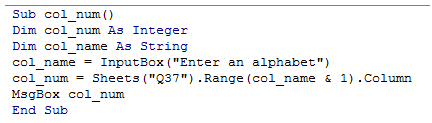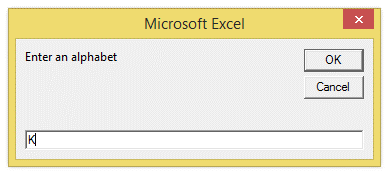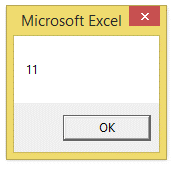## Vba column number to cell address in Excel

This is the inverse of the previous chapter, that is, to extract column number from Range address.

This scenario arises, when column number of a particular cell containing a particular data is required.

This requirement is most common in some applications, which requires the column number of a particular address.

The excel has a way to achieve this too, as explained below.

The following example demonstrates this

1. Sub col_num()
2. Dim col_num As Integer
3. Dim col_name As String
4. col_name = InputBox("Enter an alphabet")
5. col_num = Sheets("Q37").Range(col_name & 1).Column
6. MsgBox col_num
7. End Sub

The above example obtains a column name alphabet from the user and converts it to the corresponding column number.

Make sure to enter alphabet only, else, it will result in an error.

The screenshot of the editor is as shown below:The result of the above example is as shown below: